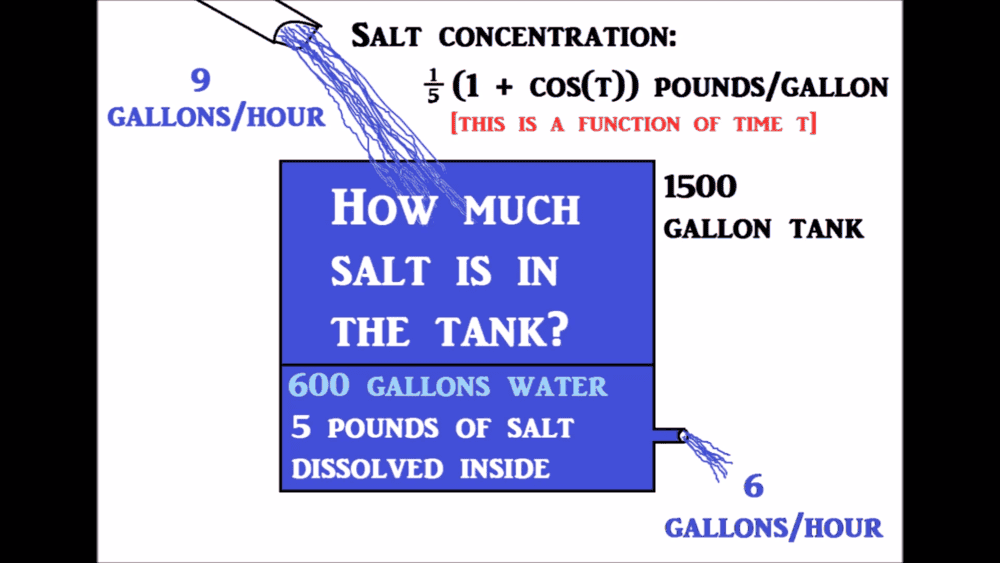# [Differential equations] Mixing problem.

Muskyboi
Homework Statement:
9 gallons/hour entering with a salt concentration of 1/5(1+cos(t)) pounds/gallon (this is a function of time t).

15000 gallon capacity tank with 600 gallons of water with 5 pounds of salt dissolved inside.

6 gallons/hour leaving.

How much salt is the tank just before it overflows?
Relevant Equations:
salt concentration= 1/5(1+cos(t)) pounds/gallon (this is a function of time t)v(t)=600+(9-6)t
=600+3t

1500=600+3t
therefore t=300 hrs when tank is full

Cin=1/5(1 + cost)

ds/dt=Rate in - rate out = CinRin - Cout*S(t)/V(t)
=1/5(1 + cost)*9 - 6*S(t)/(600+3t)

S(0)=5 ib

Solving the first order linear ODE we get: https://www.desmos.com/calculator/l7iixzgyll

therefore S(300)=279.797 Ib

does my solution look right?

Last edited:

Homework Helper
Gold Member
2022 Award
The differential equation looks right. how did you solve it?

It's a strange problem. After 300 hours the initial concentration is largely irrelevant. The average of the cosine function is 0, so the average concentration entering is ##1/5##. You would expect the final concentration to be close to this. Hence 300lb of salt, approximately.

Muskyboi
The differential equation looks right. how did you solve it?

It's a strange problem. After 300 hours the initial concentration is largely irrelevant. The average of the cosine function is 0, so the average concentration entering is ##1/5##. You would expect the final concentration to be close to this. Hence 300lb of salt, approximately.

Yes, dividing the equation for mass of salt in the tank S(t) by the volume of water in the tank V(t) would give the concentration of salt in the tank. Doing so shows that the concentration approaches 1/5 as time increases.